AP State Syllabus AP Board 9th Class Maths Solutions Chapter 13 Geometrical Constructions InText Questions and Answers.

## AP State Syllabus 9th Class Maths Solutions 13th Lesson Geometrical Constructions InText QuestionsTry These

Question
Observe the sides, angles and diagonals of quadrilateral BEFD. Name the figures given below and write properties of figures. [Page No. 283]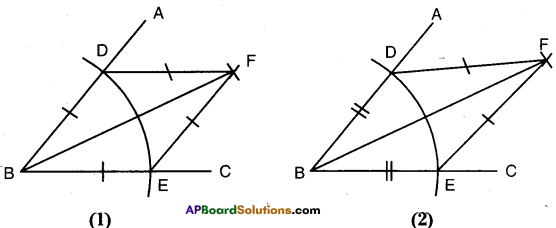Solution:
In fig. (1)
$$\overrightarrow{\mathrm{BF}}$$ is the bisector of ∠B and ∠F.
BE = BD = DF = EF
It is a rhombus

In fig. (2)
BD = BE
FD = FE
∴ BEFD is a kite.
BF is bisector of ∠B and ∠F.Try This

Question
Draw a circle, identify a point on it. Cut arcs on the circle with the length of the radius in succession. How many parts can the circle be divided into ? Give reasons. [Page No. 284]
Solution: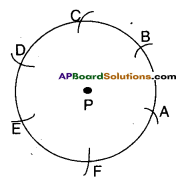Let P be the centre of the circle.
A is any point on its circumference.
It can be divided into 2π parts
∴ $$\frac{\text { Circumference }}{\text { Radius }}=\frac{2 \pi r}{r}=2 \pi$$Think, Discuss and Write

Question
Can you construct a triangle ABC with BC = 6 cm, ∠B = 60° and AB + AC = 5 cm? If , not, give reasons. (Page No. 286)
Solution:
We can’t construct a triangle with measures ∠B = 60°; BC = 6 cm and AB + AC = 5 cm.
∵ AB + AC < BC
Sum of any two sides of a triangle must be greater than the third side.Think, Discuss and Write

Question
Can you construct the triangle ABC with the same measures by changing the base angle ∠C instead of ∠B ? Draw a rough sketch and construct it.
BC = 4.2cm. ∠C = 30°, AB – AC = 1.6 cm (Page No. 287)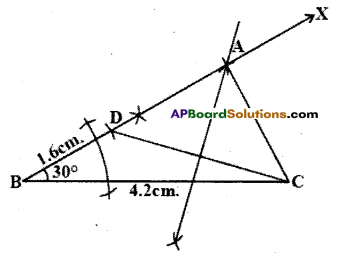Solution: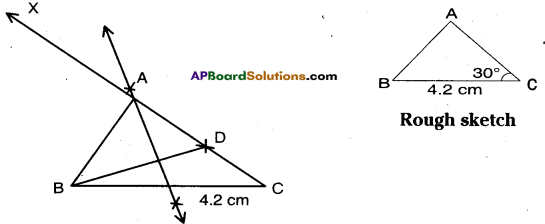Steps of construction:

1. Construct ΔBCD where BC = 4.2 cm and ∠C = 30° and AC – AB = 1.6 cm.
2. Draw perpendicular bisector of BD which meets $$\overline{\mathrm{CD}}$$ produced at A.
3. Join B, D.
4. ΔABC is the required triangle.Try These

Can you draw the triangle with the same measurements as shown in the figure in alternate way ? (Page No. 289)
[Measurements : ∠B = 6Q°, ∠C = 45° and AB + BC + CA =11 cm]
[Hint: Take ∠YXL = 60°/2 = 30° and ∠XYM = 45°/2 = 22 $$\frac { 1 }{ 2 }$$ ]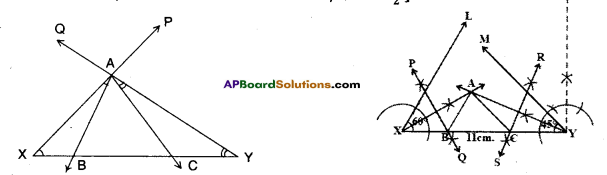Solution:
→ Draw XY = 11 cm [AB + BC + CA = 11 cm]
Construct ∠YXP = 30° at X $$\left[\frac{B}{2}=\frac{60^{\circ}}{2}=30^{\circ}\right]$$
Construct ∠XYQ = 22$$\frac { 1 }{ 2 }$$ at Y $$\left[\frac{\mathrm{C}}{2}=\frac{45^{\circ}}{2}=22 \frac{1}{2}^{\circ}\right]$$
→ $$\overrightarrow{\mathrm{XP}}$$ and $$\overrightarrow{\mathrm{YQ}}$$ meet at A.
→ At A, draw $$\overrightarrow{\mathrm{AB}}$$ such that ∠XAB = 30° where B is a point on XY.
→ Also draw $$\overrightarrow{\mathrm{AC}}$$ such that ∠YAC = 22$$\frac{1}{2}^{\circ}$$ where C is a point on XY.
→ Δ ABC is the required triangle.Try These

Question
What happens if the angle in the. circle segment is right angle ? What kind of segment do you obtain ? Draw the figure and give reason. [Page No. 290]
Solution:
If the angle in the circle segment is right angle i.e., 90°, then the angle subtended by it at the centre is 2 x 90° = 180°
Thus the line segment becomes the diameter and the circle segment becomes the semi-circle.